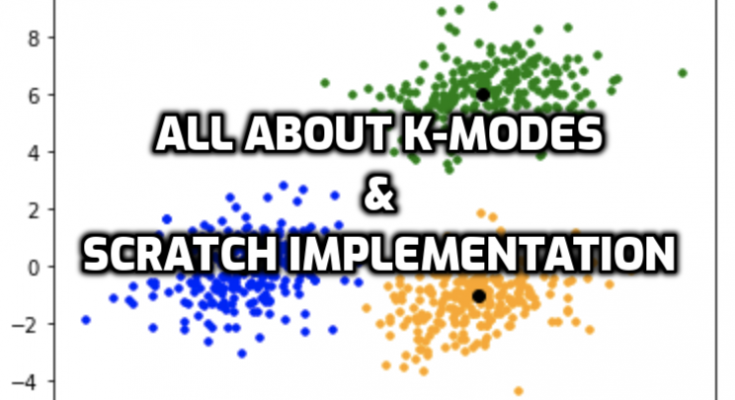# K-Modes Clustering Algorithm: Mathematical & Scratch ImplementationWelcome to the new tutorial session on “Machine Learning From Scratch.” In our previous article, we covered the K-means algorithm. This tutorial will cover the K-mode clustering algorithm from Scratch with a mathematical implementation example.

### K-MODE CLUSTERING ALGORITHM

Before entering the tutorial on k-modes, let’s revisit the k-means clustering algorithm. K-means is an unsupervised partitional clustering algorithm based on grouping data into k-numbers of clusters by determining the centroid using the Euclidean or Manhattan method for distance calculation. It updates or calculates the new centroid by calculating the mean of the data points in that cluster. We repeat the process for several iterations until successive iterations cluster data items into the same group.

Like K-means, k-mode clustering is also an unsupervised algorithm for categorical variables. This algorithm groups data points in clusters based on the number of matching categories between centroid and observation data points. Unmatched data points are counted as +1, and similar data points are counted as zero. Centroids are updated based on the maximum frequency of the categorical value, i.e., mode.

### THEORY ?? WE PREFER EXAMPLE

Let’s kick off K-Means Clustering Scratch with a simple example. Suppose we have categorical data, which should be clustered into two groups. Let’s suppose, in column 1 (C1), we have categories (M, N, O); in column 2 (C2), we have categories (P, Q, and R); and in column 3 (C3), we have categories (W, X, Y, Z). The categorical distribution of the dataset is shown in the table below:

Step 1: It is already defined that k = 2 for this problem

Step-2:  Since k = 2, we randomly select two centroids as S.N = 2 & 6, i.e. (M,Q,Z) & (N,P,X).

Step 3: In k-means, we calculate the distance of each point to each centroid using the Euclidean distance calculation method (see here). But it’s different in the case of k-modes. We compare each data point to the centroid of each observation data point. If the elements aren’t equal, we add one (+1) in each unmatching case. But, if the elements aren’t equal, we add zero, i.e., we do nothing.

Here, we are comparing the first data points with centroid 1. Since the third column doesn’t match, the total distance point is 1.

Here, we are comparing the first data points with centroid 2. Since the first & the second columns don’t match, the total distance point is 2.

Similarly, we have calculated and completed the data point table. Data points are assigned to the closest centroid with a minimum difference value.

The table shows that data points 1, 2, 4, & 5 are assigned to cluster 1st, and the rest are assigned to the second cluster.

Step 4: Here comes the actual use of modes in the algorithm. The maximum categorical occurrence (mode) is assigned to the updated centroid elements of each cluster.

We have obtained the new cluster centroids as (M, Q, X) and (N, P, Y). We repeat the same process from step 3 until successive iterations cluster data items into the same group.

### FINAL CLUSTER: K-MODES CLUSTERING

After repeating steps 3 & 4 three times i.e. after the 3rd Iteration, we get the cluster as:-

### CONCLUSION

The k-mode clustering algorithm is an extension of the k-means clustering algorithm. The k-means algorithm is the most widely used center-based partitional clustering algorithm. Huang extends the k-means clustering algorithm to the k-modes clustering algorithm to group the categorical data. The modifications done to the k-means are

• (i) using a simple matching dissimilarity measure for categorical objects,
• (ii) replacing means of clusters by modes, and
• (iii) using a frequency-based method to update the modes.

## Key Points to Remember:

• 1. K-Modes is well-suited for datasets with categorical attributes.
• 2. It uses a different distance metric (simple matching distance) compared to K-Means.
• 3. Initialization can impact the final clustering results, and different strategies can be employed.
• 4. Like K-Means, K-Modes is sensitive to the choice of K and the number of clusters. You may need to experiment with different K values and use validation metrics like the silhouette score or elbow method to determine the optimal K.

K-Modes has found applications in various domains, including market segmentation, customer profiling, text mining, and more, where categorical attributes are prevalent. However, it’s crucial to consider the impact of initialization, the choice of K, and the assessment of clustering quality when applying K-Modes in practice. Additionally, as with any clustering algorithm, the interpretability and meaningfulness of the clusters depend on the nature of the data and the problem at hand.

In summary, K-Modes is a clustering algorithm designed for categorical data that iteratively assigns data points to clusters based on categorical attribute similarity, updating cluster centroids until convergence to create distinct clusters of categorical data.